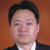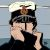# mapReduce no output into result

MALTES Corto shared this question ago

hello, how do I get a result in the window when using the function mapReduce?

MySample : I have no output

var mapFunc = () => {

emit(this.Name, 1);

};

var reduceFunc = (key, values) => {

return Array.sum(values);

};

db.MyCollection.mapReduce( mapFunc, reduceFunc,{out: { "inline":1 }});

thx```var mapFunc =function() { //Arrow functions are anonymous and change the way this binds in functions.

emit(this.Name, 1);
};

var reduceFunc = function(key, values) {
return Array.sum(values);
};

db.MyCollection.mapReduce( mapFunc, reduceFunc,{out: { "inline":1 }});```

## Replies (2)2

```var mapFunc =function() { //Arrow functions are anonymous and change the way this binds in functions.

emit(this.Name, 1);
};

var reduceFunc = function(key, values) {
return Array.sum(values);
};

db.MyCollection.mapReduce( mapFunc, reduceFunc,{out: { "inline":1 }});```1

perfect it works, thank you